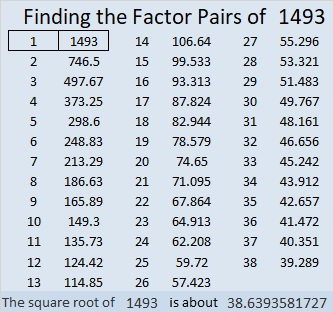# 1493 and Level 4

### Today’s Puzzle:

Write the numbers from 1 to 12 in both the first column and the top row so that those numbers are the factors of the clues given in the puzzle:### Factors of 1493:

• 1493 is a prime number.
• Prime factorization: 1493 is prime.
• 1493 has no exponents greater than 1 in its prime factorization, so √1493 cannot be simplified.
• The exponent in the prime factorization is 1. Adding one to that exponent we get (1 + 1) = 2. Therefore 1493 has exactly 2 factors.
• The factors of 1493 are outlined with their factor pair partners in the graphic below.How do we know that 1493 is a prime number? If 1493 were not a prime number, then it would be divisible by at least one prime number less than or equal to √1493. Since 1493 cannot be divided evenly by 2, 3, 5, 7, 11, 13, 17, 19, 23, 29, 31, or 37, we know that 1493 is a prime number.

### Other Facts about the number 1493:

1493 is the last prime number in the fourth prime quintuplet,
(1481, 1483, 1487, 1489, 1493), which is the smallest prime quintuplet that is not also part of a prime sextuplet.
In prime quintuplets, the first three numbers, the middle three numbers, and the last three numbers each form a prime triplet. Thus,1493 is the last prime number in the third prime triplet formed from the numbers in the fourth prime quintuplet.

1493 is the sum of two squares:
38² + 7² = 1493

1493 is the hypotenuse of a Pythagorean triple:
532-1395-1493, calculated from 2(38)(7), 38² – 7², 38² + 7²

Here’s another way we know that 1493 is a prime number: Since its last two digits divided by 4 leave a remainder of 1, and 38² + 7² = 1493 with 38 and 7 having no common prime factors, 1493 will be prime unless it is divisible by a prime number Pythagorean triple hypotenuse less than or equal to √1493. Since 1493 is not divisible by 5, 13, 17, 29, or 37, we know that 1493 is a prime number.